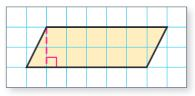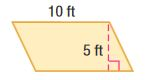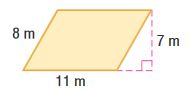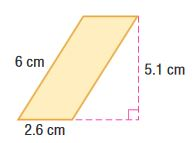Homework Explained - Math Practice 101Dear guest, you are not a registered member. As a guest, you only have read-only access to our books, tests and other practice materials.

As a registered member you can:

Registration is free and doesn't require any type of payment information. Click here to Register.
Go to page:
Chapter 9:Area;Lesson 1: Area of Parallelograms

### Guided Practice

Find the area of each parallelogram.

• Question 1•  $$\text{ units}^2.$$
• Question 2•  $$\text{ ft}^2$$
• Question 3•  $$\text{ m}^2$$
• Question 4
Find the height of a parallelogram if its base is 35 centimeters and its area is 700 square centimeters.
•  h = $$\text{ cm}$$
• Question 5

The size of the parallelogram piece in a set of tangrams is shown at the right. Find the area of the piece.•  $$\text{ cm}^2$$
• Question 6
Building on the Essential Question How are parallelograms related to triangles and rectangles?

Yes, email page to my online tutor. (if you didn't add a tutor yet, you can add one here)